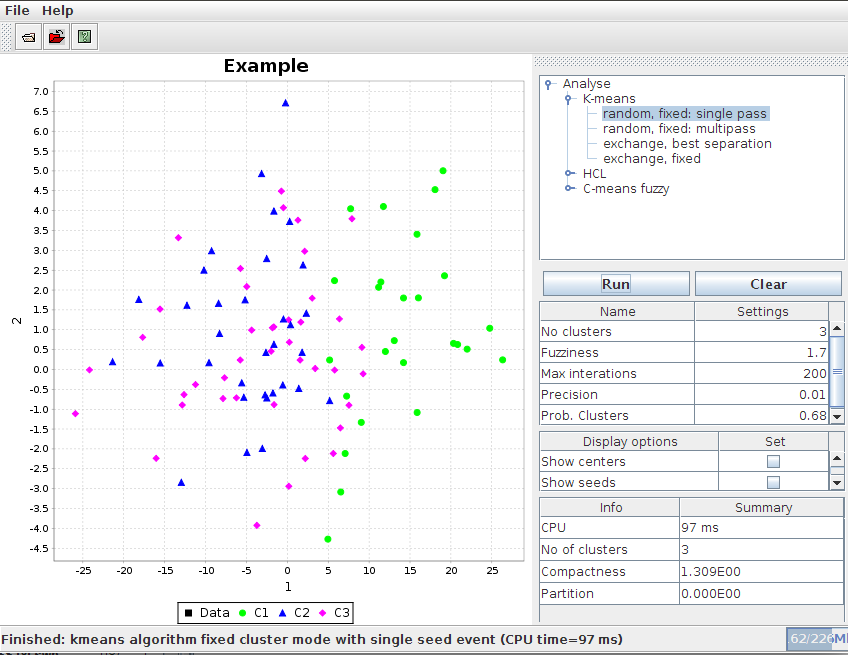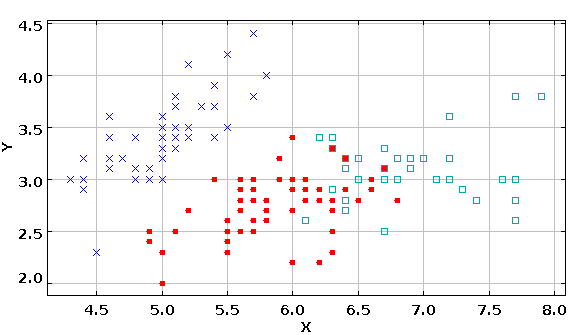# DMelt:AI/Data Clustering

Limitted access. First login to DataMelt if you are a full DataMelt member. Then login to HandWiki as a user.

# Data clustering

Cluster analysis is the task of grouping a set of objects in such a way that objects in the same group (called a cluster) are more similar to each other than to those in other groups. DataMelt contains a framework for clustering analysis, i.e. for non-supervised learning in which the classification process does not depend on a priory information. It includes the following algorithms:

• K-means clustering analysis (single and multi pass)
• C-means (fuzzy) algorithm
• Agglomerative hierarchical clustering

All algorithms can be run in a fixed cluster mode and for a best estimate, i.e. when the number of clusters is not a priory given but is found after estimation of the cluster compactness. The data points can be defined in multidimensional space.

Data clustering is based on jMinHep package. You can run this in a completely stand-alone mode, without DataMelt. DataMelt integrates this Java program and enable Java scripting.

## Using GUI

The easiest approach is to run a GUI editor to perform clustering. In the example below, we create several clusters in 3D and then passed the data holder to a GUI for clustering analysis:

This brings up a GUI editor which will run a selected algorithm:# Data clustering in JSAT

The packagejsat.clustering/ provides another Java classes for data clustering. Here is an example that uses IRIS input data, runs multiple k-means algorithms, prints scores. Then it shows the clusters on a canvas:

The canvas with the output (shown in 2D, for index 0 and 1) is:Note that IRIS data are multidimensional data and cannot be easily visualized.

# Using Jython scripts

Alternatively, one can run any clustering algorithm in batch mode without GUI. You can use Java, or any scripting programming language.

We show below a code which creates a data sample in 3D and then runs several clustering algorithms in one go. You can optionally print positions of the clusters and membership of the data points. The following modes will be used:

• K-means algorithm fixed cluster mode with single seed event
• K-means algorithm for multiple iterations
• K-means clustering using exchange method for best estimate
• K-means clustering using exchange method
• Hierarchical clustering algorithm
• Hierarchical clustering algorithm, best estimate

The output of the above script is shown below:

test 0
algorithm: kmeans algorithm fixed cluster mode with single seed event
Compactness: 1.98271418832
No of final clusters: 3
test= 1
algorithm: kmeans algorithm for multiple iterations
Compactness: 1.31227526642
No of final clusters: 3
test= 2
algorithm: K-means clustering using exchange method for best estimate
Compactness: 1.35529140568
No of final clusters: 5
test= 3
algorithm: K-means clustering using exchange method
Compactness: 1.35529140568
No of final clusters: 5
test= 4
algorithm: Hierarchical clustering algorithm
Compactness: 1.41987639705
No of final clusters: 5
test= 5
algorithm: Hierarchical clustering algorithm, best estimate
Compactness: 1.20134128248
No of final clusters: 6


You can print centers of clusters as:

Centers = pat.getCenters()
for i in range(Centers.getSize()):
g=Centers.getRow(i)
n=g.getDimension()
print i, " ",  g.getAttribute(0),g.getAttribute(1),g.getAttribute(2)

Where "Centers" are "DataHolder" container in the above example.